﻿ 异常检测——从经典算法到深度学习》14 对于流数据基于 RRCF 的异常检测

### 异常检测——从经典算法到深度学习》14 对于流数据基于 RRCF 的异常检测

2021-11-28 18:13:38

# 《异常检测——从经典算法到深度学习》

• 0 概论
• 1 基于隔离森林的异常检测算法
• 2 基于LOF的异常检测算法
• 3 基于One-Class SVM的异常检测算法
• 4 基于高斯概率密度异常检测算法
• 5 Opprentice——异常检测经典算法最终篇
• 6 基于重构概率的 VAE 异常检测
• 7 基于条件VAE异常检测
• 8 Donut: 基于 VAE 的 Web 应用周期性 KPI 无监督异常检测
• 9 异常检测资料汇总（持续更新&抛砖引玉）
• 10 Bagel: 基于条件 VAE 的鲁棒无监督KPI异常检测
• 12 Buzz: 对复杂 KPI 基于VAE对抗训练的非监督异常检测
• 14 对于流数据基于 RRCF 的异常检测

• VAE 模型基本原理简单介绍
• GAN 数学原理简单介绍以及代码实践

# 14. 对于流数据基于 RRCF 的异常检测

2016 Robust random cut forest based anomaly detection on streams

RRCF 的实现论文 下载地址

## 14.1 简要概述论文内容

### 14.1.1 核心思想与方法

• 什么是RRCF？
• RRCF 相对于 RF 改进之处在哪，怎么改进的？
• RRCF 是如何应用在流数据（即时间序列数据）的异常检测的。

### 14.1.2 RRCF 的生成过程

• 每棵树的生成是互不相干的；
• 每棵树生成对应的数据的采样也是互不相关的（有放回地随机采样）
• 每棵树的地位都是相等的，这个与boosting思想差别很大。

1. 选择一个与 ℓ i ∑ j ℓ j \frac{\ell_i}{\sum_j \ell_j} 成比例的随机维度，其中 ℓ i = m a x x ∈ S x i − m i n x ∈ S x i \ell_i = max_{x\in S}x_i-min_{x\in S}x_i
2. 选一个 X i ∼ U n i f o r m [ m i n x ∈ S x i , m a x x ∈ S x i ] X_i \sim Uniform[min_{x\in S} x_i, max_{x\in S}x_i] 也就是从最小到最大的均匀分布。
3. S 1 = { x ∣ x ∈ S , x i ≤ X i } S_1=\{x|x\in S,x_i\le X_i\} ​​​​​ ， S 2 = S     S 1 S_2=S\ \text{\\}\ S_1 ​​​​​ 和 基于 S 1 S_1 S 2 S_2 的递归。

### 14.1.3 RRCF 的分割过程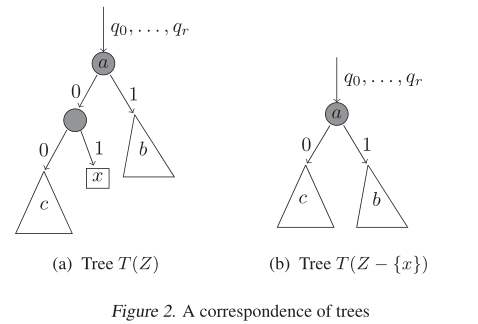### 14.1.4 定义异常

D I S P ( x , Z ) = ∑ T , y ∈ Z − { x } P r [ T ] ( f ( y , Z , T ) − f ( y , Z − { x } , T ′ ) ) DISP(x, Z)=\sum_{T, y\in Z-\{x\}} \mathbb{P}r[T](f(y,Z,T)-f(y,Z-\{x\},T'))

1The converse is not true, this is a many-to-one mapping.

### 14.1.5 基于流的森林维护

• 对于任何维度 i i ，使用加权隔离林算法选择维度 i i 中分裂的轴平行切割的概率与选择分裂的轴平行切割的条件概率完全相同 S ∪ { p } S\cup \{p\} ​ ，条件是不将 p p S S 的所有点隔离。
• 给定 R R C F ( S ) RRCF(S) 的随机树 S ∪ { p } S\cup \{p\} ，条件是第一次切割将 p p S S 的所有点隔离，树的其余部分是 R R C F ( S ) RRCF(S) 中的随机树。

## 14.2 动手实验

### 14.2.1 环境安装

\$ pip install rrcf


RRCF 依赖包括：

• numpy (>= 1.15)

RRCF 官方提供的例子的依赖包括：

• pandas (>= 0.23)
• scipy (>= 1.2)
• scikit-learn (>= 0.20)
• matplotlib (>= 3.0)

### 14.2.2 第一个例子

import numpy as np
import rrcf

# A (robust) random cut tree can be instantiated from a point set (n x d)
X = np.random.randn(100, 2)
tree = rrcf.RCTree(X)

# A random cut tree can also be instantiated with no points
tree = rrcf.RCTree()


tree = rrcf.RCTree()

for i in range(6):
x = np.random.randn(2)
tree.insert_point(x, index=i)
tree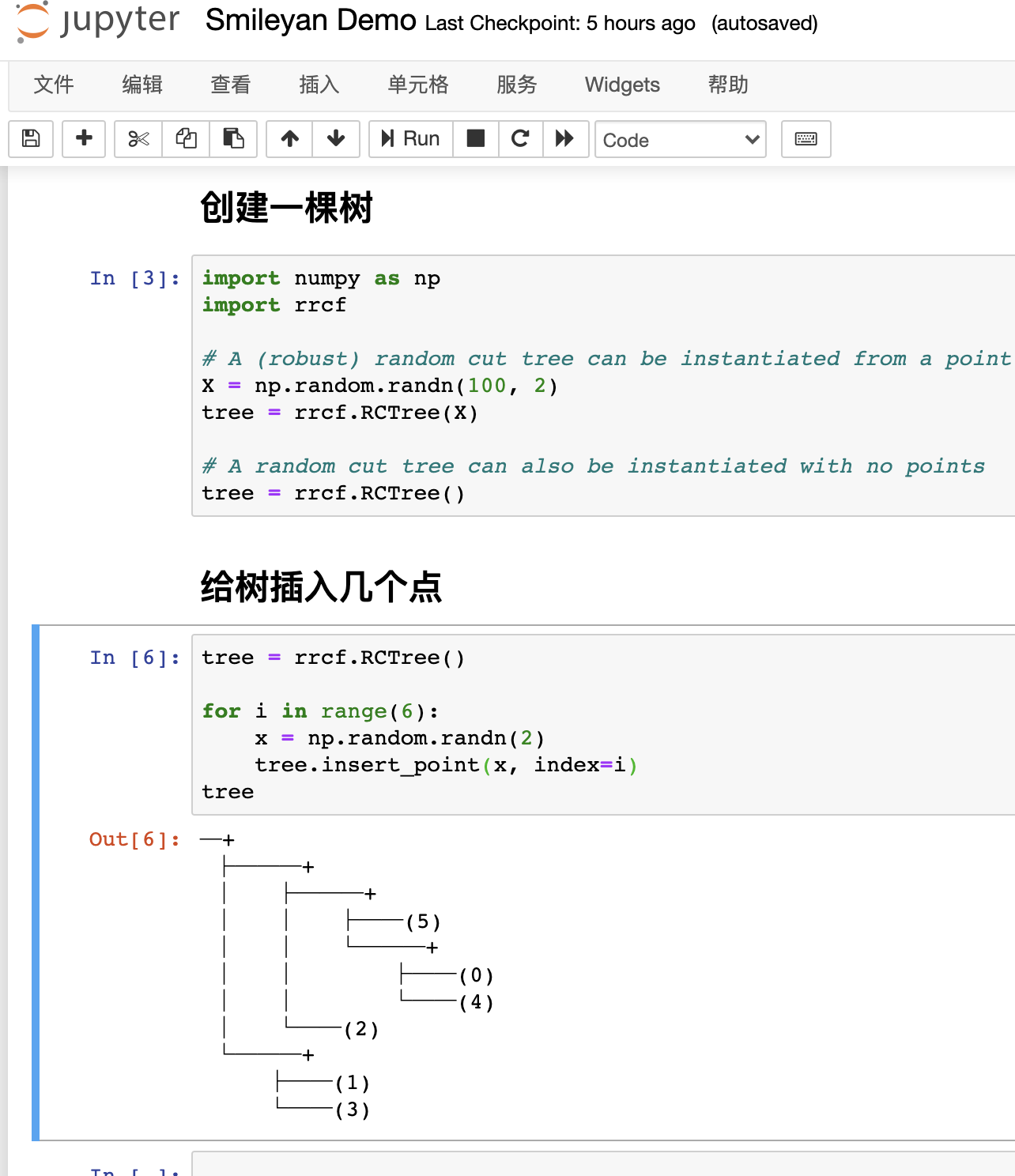tree.forget_point(2)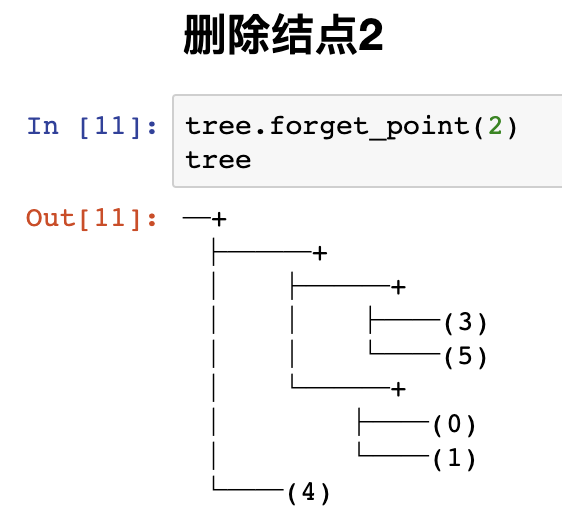### 14.2.3 RRCF用于异常检测

# Seed tree with zero-mean, normally distributed data
X = np.random.randn(100,2)
tree = rrcf.RCTree(X)

# Generate an inlier and outlier point
inlier = np.array([0, 0])
outlier = np.array([4, 4])

# Insert into tree
tree.insert_point(inlier, index='inlier')
tree.insert_point(outlier, index='outlier')


tree.codisp('inlier')

tree.codisp('outlier')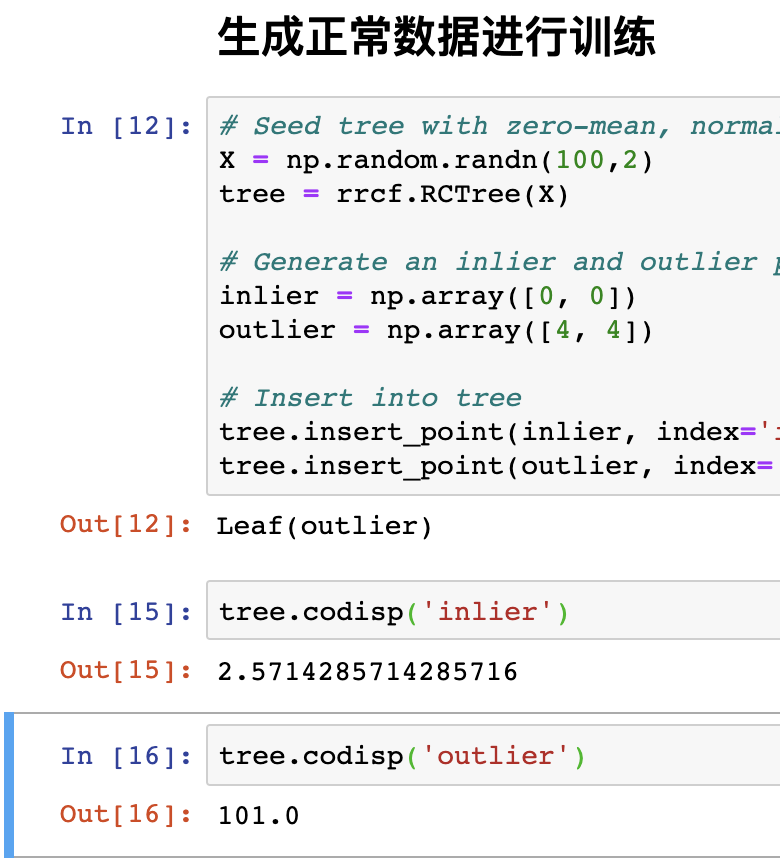### 14.2.4 RRCF 用于批次异常检测

import numpy as np
import pandas as pd
import rrcf

# Set sample parameters
np.random.seed(0)
n = 2010
d = 3

# Generate data
X = np.zeros((n, d))
X[:1000,0] = 5
X[1000:2000,0] = -5
X += 0.01*np.random.randn(*X.shape)

# Set forest parameters
num_trees = 100
tree_size = 256
sample_size_range = (n // tree_size, tree_size)

# Construct forest
forest = []
while len(forest) < num_trees:
# Select random subsets of points uniformly
ixs = np.random.choice(n, size=sample_size_range,
replace=False)
# Add sampled trees to forest
trees = [rrcf.RCTree(X[ix], index_labels=ix)
for ix in ixs]
forest.extend(trees)

# Compute average CoDisp
avg_codisp = pd.Series(0.0, index=np.arange(n))
index = np.zeros(n)
for tree in forest:
codisp = pd.Series({leaf : tree.codisp(leaf)
for leaf in tree.leaves})
avg_codisp[codisp.index] += codisp
avg_codisp /= index


from mpl_toolkits.mplot3d import Axes3D
import matplotlib.pyplot as plt
from matplotlib import colors

threshold = avg_codisp.nlargest(n=10).min()

fig = plt.figure(figsize=(12,4.5))
sc = ax.scatter(X[:,0], X[:,1], X[:,2],
c=np.log(avg_codisp.sort_index().values),
cmap='gnuplot2')
plt.title('log(CoDisp)')
sc = ax.scatter(X[:,0], X[:,1], X[:,2],
linewidths=0.1, edgecolors='k',
c=(avg_codisp >= threshold).astype(float),
cmap='cool')
plt.title('CoDisp above 99.5th percentile')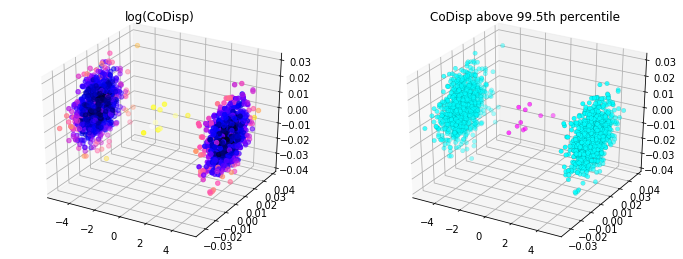### 14.2.5 RRCF 用于流数据异常检测

import numpy as np
import rrcf

# Generate data
n = 730
A = 50
center = 100
phi = 30
T = 2*np.pi/100
t = np.arange(n)
sin = A*np.sin(T*t-phi*T) + center
sin[235:255] = 80

# Set tree parameters
num_trees = 40
shingle_size = 4
tree_size = 256

# Create a forest of empty trees
forest = []
for _ in range(num_trees):
tree = rrcf.RCTree()
forest.append(tree)

# Use the "shingle" generator to create rolling window
points = rrcf.shingle(sin, size=shingle_size)

# Create a dict to store anomaly score of each point
avg_codisp = {}

# For each shingle...
for index, point in enumerate(points):
# For each tree in the forest...
for tree in forest:
# If tree is above permitted size, drop the oldest point (FIFO)
if len(tree.leaves) > tree_size:
tree.forget_point(index - tree_size)
# Insert the new point into the tree
tree.insert_point(point, index=index)
# Compute codisp on the new point and take the average among all trees
if not index in avg_codisp:
avg_codisp[index] = 0
avg_codisp[index] += tree.codisp(index) / num_trees



import pandas as pd
import matplotlib.pyplot as plt
import seaborn as sns

fig, ax1 = plt.subplots(figsize=(10, 5))

color = 'tab:red'
ax1.set_ylabel('Data', color=color, size=14)
ax1.plot(sin, color=color)
ax1.tick_params(axis='y', labelcolor=color, labelsize=12)
ax1.set_ylim(0,160)
ax2 = ax1.twinx()
color = 'tab:blue'
ax2.set_ylabel('CoDisp', color=color, size=14)
ax2.plot(pd.Series(avg_codisp).sort_index(), color=color)
ax2.tick_params(axis='y', labelcolor=color, labelsize=12)
ax2.grid('off')
ax2.set_ylim(0, 160)
plt.title('Sine wave with injected anomaly (red) and anomaly score (blue)', size=14)



Smileyan
2021.11/28 18:09

 论文地址
 源码地址